# Backpropagation.pptx

12 Dec 2022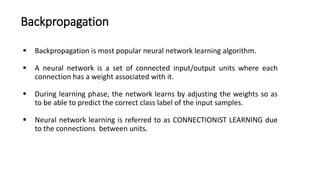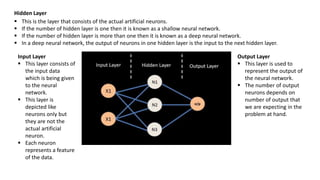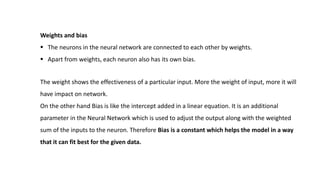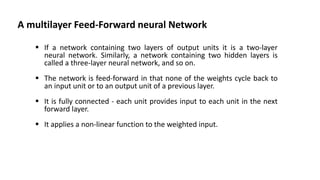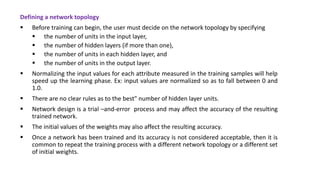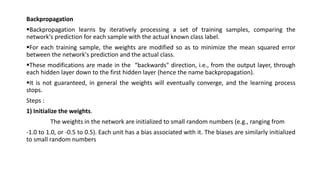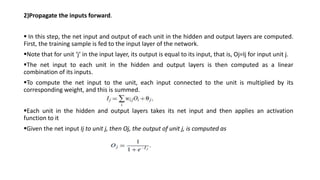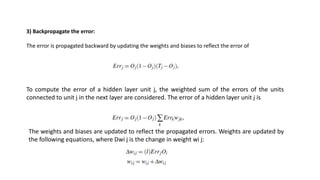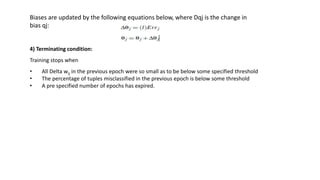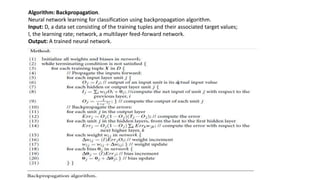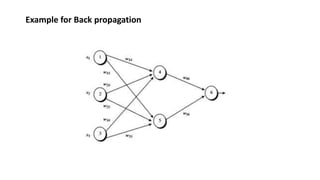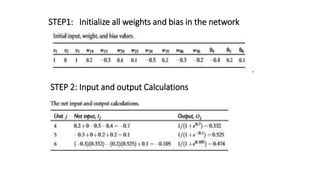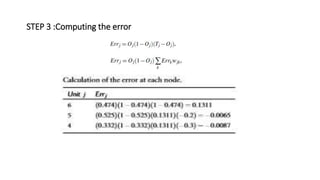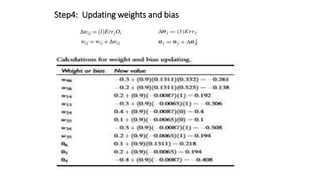1 sur 15

### Backpropagation.pptx

• 2. Backpropagation  Backpropagation is most popular neural network learning algorithm.  A neural network is a set of connected input/output units where each connection has a weight associated with it.  During learning phase, the network learns by adjusting the weights so as to be able to predict the correct class label of the input samples.  Neural network learning is referred to as CONNECTIONIST LEARNING due to the connections between units.
• 3. Input Layer  This layer consists of the input data which is being given to the neural network.  This layer is depicted like neurons only but they are not the actual artificial neuron.  Each neuron represents a feature of the data. Hidden Layer  This is the layer that consists of the actual artificial neurons.  If the number of hidden layer is one then it is known as a shallow neural network.  If the number of hidden layer is more than one then it is known as a deep neural network.  In a deep neural network, the output of neurons in one hidden layer is the input to the next hidden layer. Output Layer  This layer is used to represent the output of the neural network.  The number of output neurons depends on number of output that we are expecting in the problem at hand.
• 4. The weight shows the effectiveness of a particular input. More the weight of input, more it will have impact on network. On the other hand Bias is like the intercept added in a linear equation. It is an additional parameter in the Neural Network which is used to adjust the output along with the weighted sum of the inputs to the neuron. Therefore Bias is a constant which helps the model in a way that it can fit best for the given data. Weights and bias  The neurons in the neural network are connected to each other by weights.  Apart from weights, each neuron also has its own bias.
• 5. A multilayer Feed-Forward neural Network  If a network containing two layers of output units it is a two-layer neural network. Similarly, a network containing two hidden layers is called a three-layer neural network, and so on.  The network is feed-forward in that none of the weights cycle back to an input unit or to an output unit of a previous layer.  It is fully connected - each unit provides input to each unit in the next forward layer.  It applies a non-linear function to the weighted input.
• 6. Defining a network topology  Before training can begin, the user must decide on the network topology by specifying  the number of units in the input layer,  the number of hidden layers (if more than one),  the number of units in each hidden layer, and  the number of units in the output layer.  Normalizing the input values for each attribute measured in the training samples will help speed up the learning phase. Ex: input values are normalized so as to fall between 0 and 1.0.  There are no clear rules as to the best" number of hidden layer units.  Network design is a trial –and-error process and may affect the accuracy of the resulting trained network.  The initial values of the weights may also affect the resulting accuracy.  Once a network has been trained and its accuracy is not considered acceptable, then it is common to repeat the training process with a different network topology or a different set of initial weights.
• 7. Backpropagation Backpropagation learns by iteratively processing a set of training samples, comparing the network's prediction for each sample with the actual known class label. For each training sample, the weights are modified so as to minimize the mean squared error between the network's prediction and the actual class. These modifications are made in the “backwards" direction, i.e., from the output layer, through each hidden layer down to the first hidden layer (hence the name backpropagation). It is not guaranteed, in general the weights will eventually converge, and the learning process stops. Steps : 1) Initialize the weights. The weights in the network are initialized to small random numbers (e.g., ranging from -1.0 to 1.0, or -0.5 to 0.5). Each unit has a bias associated with it. The biases are similarly initialized to small random numbers
• 8. 2)Propagate the inputs forward.  In this step, the net input and output of each unit in the hidden and output layers are computed. First, the training sample is fed to the input layer of the network. Note that for unit ‘j’ in the input layer, its output is equal to its input, that is, Oj=Ij for input unit j. The net input to each unit in the hidden and output layers is then computed as a linear combination of its inputs. To compute the net input to the unit, each input connected to the unit is multiplied by its corresponding weight, and this is summed. Each unit in the hidden and output layers takes its net input and then applies an activation function to it Given the net input Ij to unit j, then Oj, the output of unit j, is computed as
• 9. 3) Backpropagate the error: The error is propagated backward by updating the weights and biases to reflect the error of To compute the error of a hidden layer unit j, the weighted sum of the errors of the units connected to unit j in the next layer are considered. The error of a hidden layer unit j is The weights and biases are updated to reflect the propagated errors. Weights are updated by the following equations, where Dwi j is the change in weight wi j:
• 10. Biases are updated by the following equations below, where Dqj is the change in bias qj: 4) Terminating condition: Training stops when • All Delta wij in the previous epoch were so small as to be below some specified threshold • The percentage of tuples misclassified in the previous epoch is below some threshold • A pre specified number of epochs has expired.
• 11. Algorithm: Backpropagation. Neural network learning for classification using backpropagation algorithm. Input: D, a data set consisting of the training tuples and their associated target values; l, the learning rate; network, a multilayer feed-forward network. Output: A trained neural network.
• 12. Example for Back propagation
• 13. STEP1: Initialize all weights and bias in the network STEP 2: Input and output Calculations
• 14. STEP 3 :Computing the error